## The Temperature of the Vacuum

Quantum field theory predicts that the temperature of empty space should depend on the observer’s motion, increasing proportionally with acceleration. Here I attempt an accessible introduction to this striking effect, related to Hawking radiation and discovered independently by Fulling, Davies, and Unruh, assuming only sophomore-level physics (including hyperbolic functions) with some assistance from Mathematica.

## Hyperbolic Motion

Constant acceleration in Newtonian mechanics is parabolic, while constant acceleration in Einsteinian mechanics is hyperbolic and asymptotic to light speed $c = 1$ in natural units. For 1+1-dimensional Minkowski spacetime, the difference in squared space and time displacements is the square of proper time displacement,

$$d\tau^2 = dt^2 - dx^2.$$

For constant proper acceleration, this has the solution

$$dt = d\tau \cosh a\tau,\\dx = d\tau \sinh a\tau,$$

with velocity

$$v = \frac{dx}{dt} = \tanh a\tau \le 1,$$

where for small times $v \sim a \tau \sim a t$. If $t = 0$ and $a x = 1$ at $\tau = 0$, then integration gives

$$a t = \sinh a \tau,\\a x = \cosh a\tau.$$

Hyperbolic identities then imply

$$a^2 x^2 - a^2 t^2 = 1$$

and

$$a x + a t = e^{a\tau}.$$## Quantum Vacuum

Due to Heisenberg indeterminacy, electromagnetic fluctuations fill the vacuum. Consider a single such sinusoidal wave of angular frequency $\omega_0 = k_0$ in natural units. If you move at constant velocity, you observe the wave doppler-shifted to a different frequency. But if you move at constant acceleration, you observe the wave doppler-shifted to a range of frequencies corresponding to your range of velocities. For an accelerated observer at time $\tau$,

$$\phi[x,t] = \exp\left[i(k_0 x + \omega_0 t)\right] = \exp\left[i\omega_0(x + t)\right] = \exp\left[{i \frac{\omega_0}{a} e^{a \tau}} \right] = \phi[\tau].$$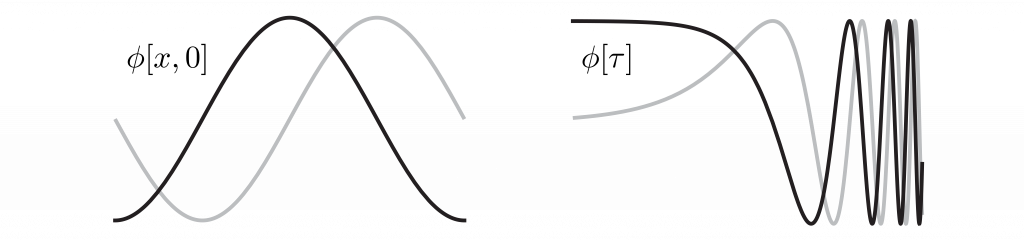Expand this waveform as a sum of harmonics

$$\phi[\tau] = \int_{-\infty}^{\infty}\,\frac{d\omega}{2\pi} \Phi[\omega] \exp[-i \omega \tau]$$

where the Fourier components

$$\Phi[\omega] = \int_{-\infty}^{\infty}d\tau\, \phi[\tau] \exp[+i \omega \tau].$$

To regularize this divergent integral, subtract a tiny imaginary part $i \epsilon$ from the angular frequency $\omega$ to incorporate a decaying exponential factor $e^{-\epsilon \tau}$ in the integrand, and zero it after integrating. Find

$$\Phi[\omega] = \lim_{\epsilon\rightarrow 0} \int_{-\infty}^{\infty}d\tau\, \exp\left[ i \frac{\omega_0}{a} e^{a\tau} \right] \exp[+i (\omega - i \epsilon) \tau]\\~ \\= \lim_{\epsilon\rightarrow 0}\, \exp\left[ i\frac{\pi}{2} \left(\frac{\epsilon + i\omega}{a}\right) \right]\left(\frac{a}{\omega_0}\right)^{(\epsilon + i \omega)/a}\frac{1}{a} \Gamma\left[\frac{\epsilon + i \omega}{a} \right] = \exp\left[-\frac{\pi}{2} \frac{\omega}{a}\right]\left(\frac{a}{\omega_0}\right)^{i \omega / a}\frac{1}{a} \Gamma\left[i \frac{\omega}{a} \right],$$

where $\Gamma[n+1] = n!$ analytically continues the factorial function to the complex plane. The spectrum is the absolute square of the Fourier transform,

$$S[\omega] = \left|\Phi[\omega]\right|^2 = \frac{\pi}{a\omega} \left(\coth\left[ \pi \frac{\omega}{a} \right]-1 \right) = \frac{2\pi}{\omega a} \frac{1}{e^{2\pi \omega/a} - 1} \propto \frac{1}{e^{\hbar \omega/k T}-1},$$

where the Planck factor suggests Bose-Einstein statistics and a thermal photon bath of temperature $kT = a \hbar / 2\pi$. In SI units,

$$T = \frac{a \hbar}{2\pi k c} \sim 40~\text{zK}~\left(\frac{a}{g_E} \right),$$

where $g_E$ is Earth’s surface gravity, and a zeptokelvin is very cool.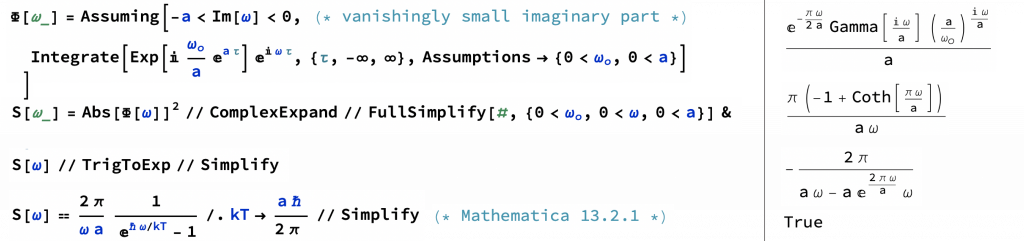## Hawking-Unruh Temperature

Just prior to the 1970s work of Fulling, Davies, and Unruh, Stephen Hawking famously predicted that despite their reputations black holes should radiate with an effective temperature

$$T = \frac{\kappa \hbar}{2\pi k c},$$

where $\kappa$ is the black hole’s surface gravity (observed at infinity). The Unruh and Hawking results may be linked by the equivalence principle, which equates acceleration and gravity, and by event horizons. The black hole horizon is a boundary that causally disconnects the interior from the exterior. Similarly, when you accelerate, a Rindler horizon appears a distance $c^2/a \sim 1~\text{ly} \left(g_E / a \right)$ behind you, causally disconnecting you from a region of spacetime whose photons you can outrun (so long as your acceleration continues).

## A Century of Compton Scattering

One hundred years ago today the Physical Review published research on light scattering electrons that would earn its author, Wooster graduate Arthur Compton, a Physics Nobel Prize.

By relativistically conserving spacetime momentum, as in the diagram below, and treating light as particles now called photons, Compton discovered that deflecting an electron through an angle $\theta$ stretches the light’s wavelength by

$$\frac{ \Delta \lambda}{\lambda_c} = 1 - \cos\theta,$$

where $\lambda_c = h / mc \sim 1/40 \textup{~\AA}$ is the Compton wavelength of the electron. Compton’s experiment helped convince the physics community of wave-particle duality. Today, sophomores Compton scatter in our Modern Physics lab.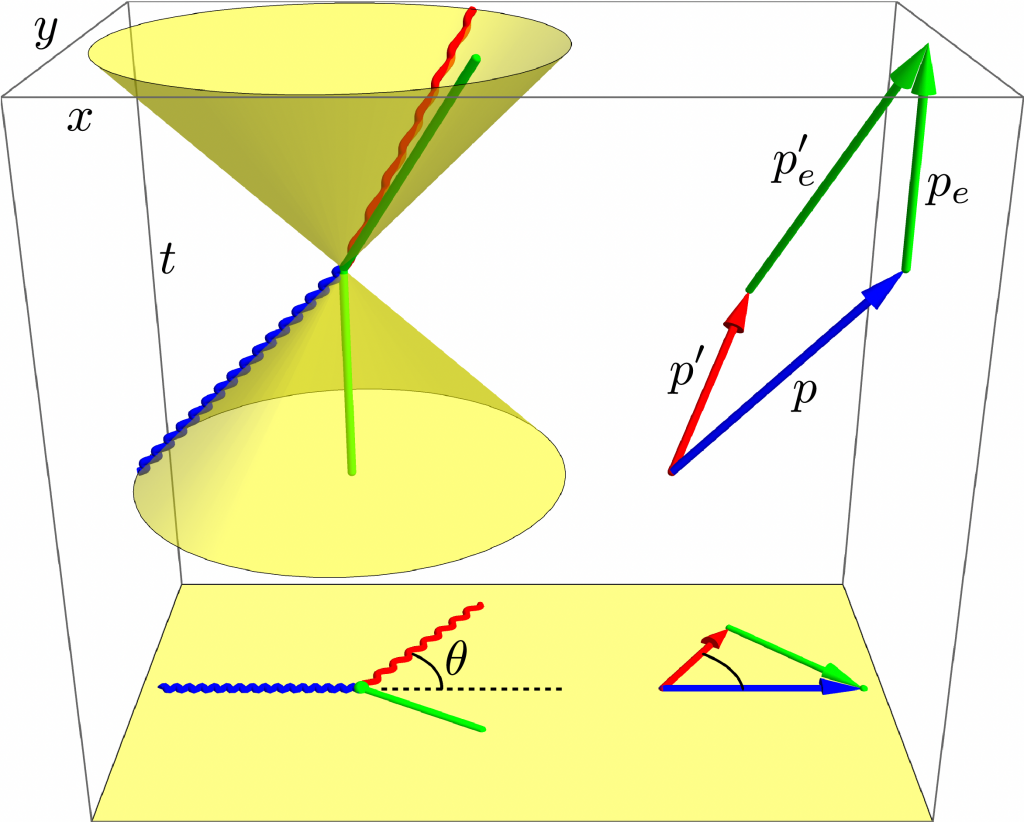Compton scattering of a photon (blue to red) from an electron (green) in space (bottom) and spacetime (top). The photon lives on the light cones while the electron lives in the light cones.

## We Are Going

After half a century confined to low-Earth orbit, and as soon as late next year, humans will once again leave Earth and voyage to Moon. The reality of this exciting adventure crystallized earlier this month when NASA announced the diverse and inspiring Artemis II crew: Clockwise from left in the photo below are Christina Koch, Victor Glover, Jeremy Hansen, and Reid Wiseman.

Christina holds the record for longest space flight by a woman, during which she participated in the first all-woman spacewalk. She is now scheduled to become the first woman to fly around Moon. Along with two electrical engineer degrees, Christina obtained a bachelor’s degree in physics from NC State, and I often think of her as I walk to my present office in NC State’s physics building (although I understand that physics moved into the renovated Riddick shortly after she graduated).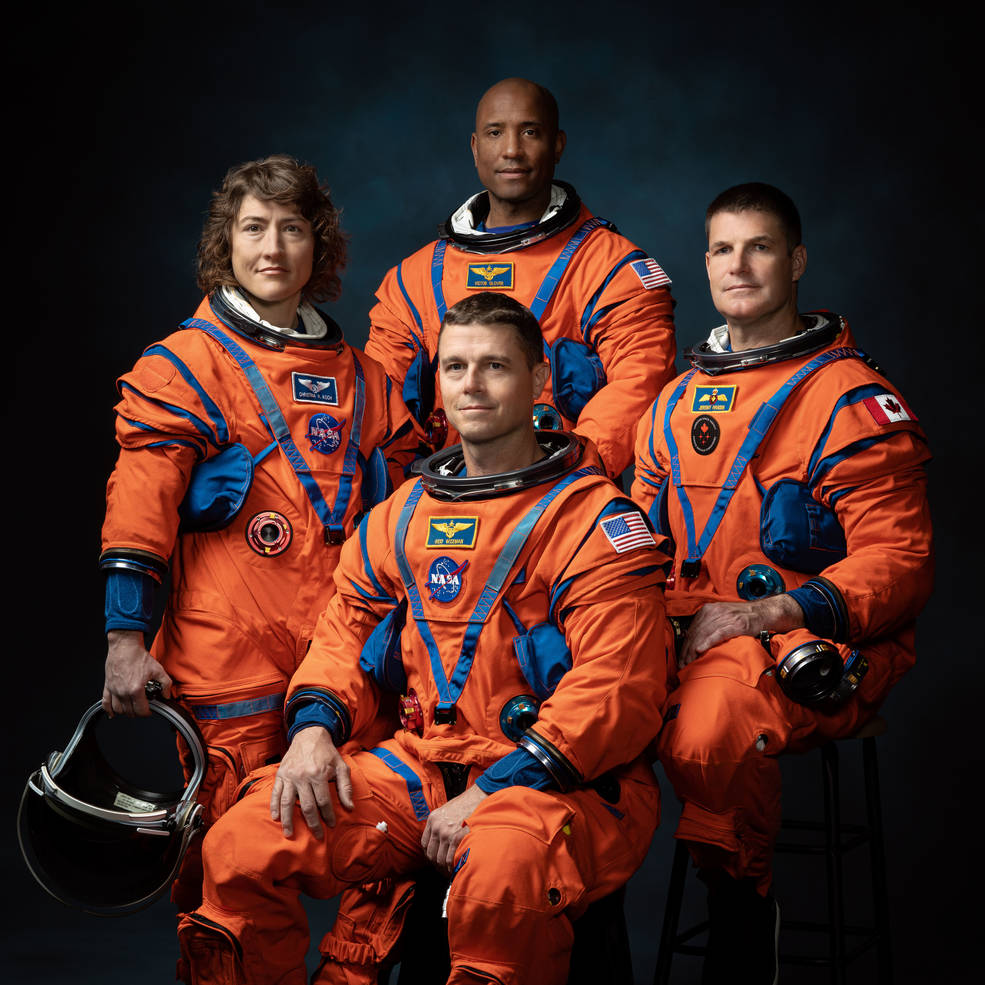Christina Koch, Victor Glover, Jeremy Hansen, Reid Wiseman, the crew of Artemis II.
(Photo by Josh Valcarcel for NASA.)

## Behold, an ein Stein!

This academic year has been thrilling: first nuclear fusion breakeven, now an ein Stein!

Last week, a preprint at arxiv.org by David Smith et al. announced an “ein Stein”, or one stone, a shape that forces a non periodic tiling of the plane, ending a half-century quest by many researchers, including me. A retiree and tiling enthusiast, Smith discovered the shape, which he calls “the hat”, and his professional colleagues provided mathematical and computational rigor.

In the 1960s, Robert Berger found a set of 20 426 tile-types that tile a plane but only non periodically — a wonderful mix of the expected and the surprising, a kind of visual music. By the 1970s, Roger Penrose has reduced that to just 2 tile-types, but a further reduction to just 1 tile-type proved elusive. Of Smith’s hat, the 91-year-old Roger Penrose reportedly observed, “It’s a really good shape, strikingly simple”, and I agree.

In the grayscale figure below, an underlying hexagonal grid emphasizes the hat’s construction. Given the prior theoretical and computational work that failed to find it, the hat is a surprisingly simple polykite formed from 8 kites or 4 double kites. In the color figure below, I color the hats according to their orientations (brightnesses) and reflections (hues), something I’ve dreamed of doing for many years.

You could order many flat paving stones shaped like the hat and translate, rotate, and flip them to non periodically tile your patio. I just might.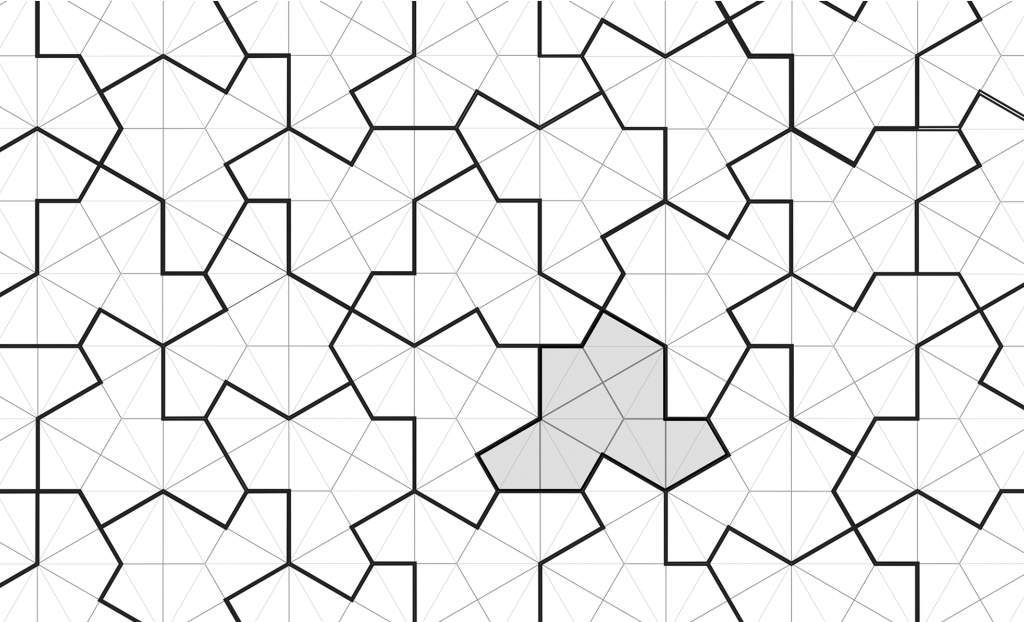Figure 1.1 from David Smith et al. “An aperiodic monotile” emphasizing the underlying hexagonal foundation of “the hat”, which is a tridecagon made from 8 kites or 4 double kites.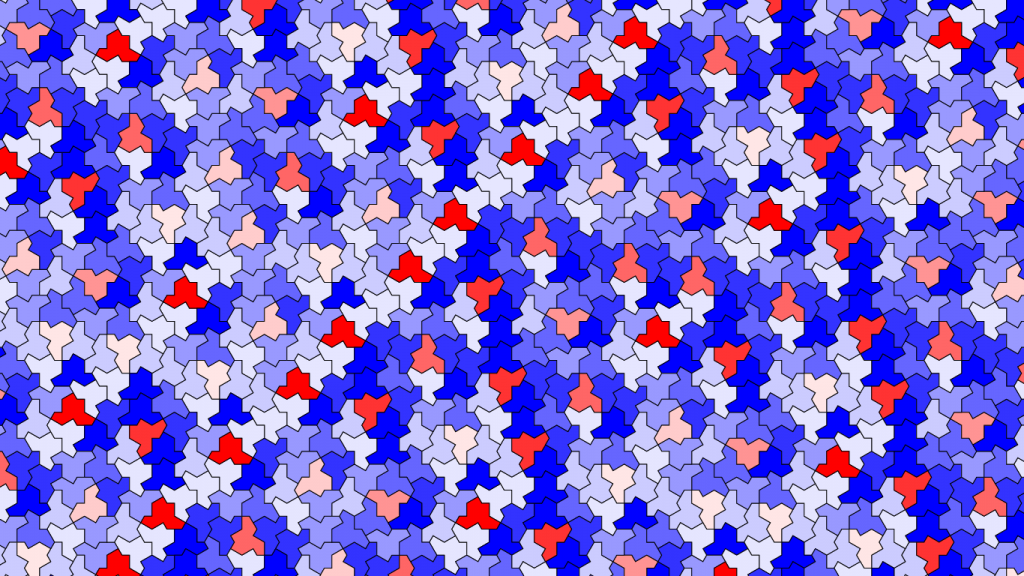The hat forces a non periodic tiling of the plane, where brightnesses indicate 1 of 6 orientations and hues indicate 1 of 2 reflections. (Alternately, the orientations and reflections can all be considered rotations about axes perpendicular or parallel to the plane.)

## Generalizing Coulomb’s Law

The forces between two electric charges in arbitrary motion are complicated by velocity, acceleration, and time-delay effects. The forces need not even lie along the line joining the two charges!

Suppose a source charge $q^\prime$ is at position $\vec r^{\,\prime}$ with velocity $\vec v^{\,\prime}$ and acceleration $\vec{a}^{\,\prime}$, and a test charge $q$ is at position $\vec r$ with velocity $\vec v$ and acceleration $\vec{a}$. Let their separation $\vec{\mathcal{R}}= \vec r - \vec r^{\,\prime}$. Since electromagnetic “news” travels at light speed $\color{blue}c\color{black} = 1$ (in natural units), define the earlier retarded time $s < t$  implicitly by $t - s = \mathcal{R}[s] / \color{blue}c\color{black} = \mathcal{R}_{\color{red}s}$.

Liénard-Wiechert electric and magnetic potentials at test charge $q$ due to source charge $q^\prime$ are

$$\varphi_{\color{red}t} =\frac{q^\prime_e}{4\pi \mathcal{R}_{\color{red}s} }=\frac{q^\prime}{4\pi \mathcal{R}_{\color{red}s} } \left( \frac{1}{1 - \hat{\mathcal{R} }_{\color{red}s} \cdot \vec v^{\,\prime}_{\color{red}s}} \right), \hspace{1cm} \vec A_{\color{red}t}= \varphi^\prime_{\color{red}s} \vec v^{\,\prime}_{\color{red}s},$$

where the source charge $q^\prime$ is “smeared” to an effective charge $q^\prime_e$ by its motion, and the subscripts indicate evaluation times. If the source charge velocity $\vec v^{\,\prime}_{\color{red}s} = 0$, then the potentials simplify to the electrostatics limit. Differentiate the potentials to find Maxwell’s electric and magnetic fields

$$\vec{\mathcal{E}} = - \vec \nabla \varphi - \partial_t \vec A \hspace{1cm} \vec{\mathcal{B}} = \vec \nabla \times \vec A,$$

and the Lorentz force law

$$\vec F = q \left( \vec{\mathcal E} + \vec v \times \vec{\mathcal B}\right)$$

generalizes Coulomb’s law to

$$\vec F_{\color{red}t} = q \frac{ q^\prime } { 4 \pi \mathcal{R}^2_{\color{red}s}} \left( \frac{ 1 } {1 - \hat{\mathcal{R}}_{\color{red}s} \cdot \vec{v}^{\,\prime}_{\color{red}s} }\right)^3 \bigg( \frac{}{} (1-v^{\prime 2}_{\color{red}s})(\hat{\mathcal{R}}_{\color{red}s}-\vec{v}^{\,\prime}_{\color{red}s}) + \vec{\mathcal{R}}_{\color{red}s} \times \left((\hat{\mathcal{R}}_{\color{red}s}-\vec{v}^{\,\prime}_{\color{red}s}) \times \vec{a}^{\,\prime}_{\color{red}s} \right)$$ $$\hspace{3.4cm} + \,\vec v_{\color{red}t} \times \left( \hat{\mathcal{R}}_{\color{red}s} \times \left( (1-v^{\prime 2}_{\color{red}s})(\hat{\mathcal{R}}_{\color{red}s}-\vec{v}^{\,\prime}_{\color{red}s}) + \vec{\mathcal{R}}_{\color{red}s} \times \left((\hat{\mathcal{R}}_{\color{red}s}-\vec{v}^{\,\prime}_{\color{red}s}) \times \vec{a}^{\,\prime}_{\color{red}s} \right) \right) \right) \, \bigg).$$

If the test charge velocity $\vec v = \vec 0$, then the magnetic terms vanish; if the source charge acceleration $\vec{a}^{\,\prime} = \vec 0$, then the radiation terms vanish; if, in addition, the source charge velocity $\vec v\,^\prime = \vec 0$, then the generalized Coulomb’s law reduces to the familiar electrostatic limit. Finally, Newton’s second law with Einstein’s momentum

$$\vec F = \frac{d\vec p}{dt} = \frac{d}{dt} \frac{ m \vec v }{\sqrt{1 - v^2}} = \frac{ m \vec a}{\sqrt{1 - v^2}} + \frac{ m \vec v}{(1 - v^2)^{3/2}} \vec v \cdot \vec a$$

generates the motion equations using 3-vectors and lab time (instead of 4-vectors and proper time), succinctly summarizing all of electromagnetism.

Using Mathematica, I eliminated present time $t$ from the motion equations with the substitution $t = s + \mathcal{R}_{\color{red}s}$ and numerically integrated them with respect to the retarded time $s$ for various initial conditions, as in the figure below. Positive and negative charge pairs tugged by non-zero net forces spiral into one another as they radiate away energy and momentum, graphically demonstrating the instability of classical atoms.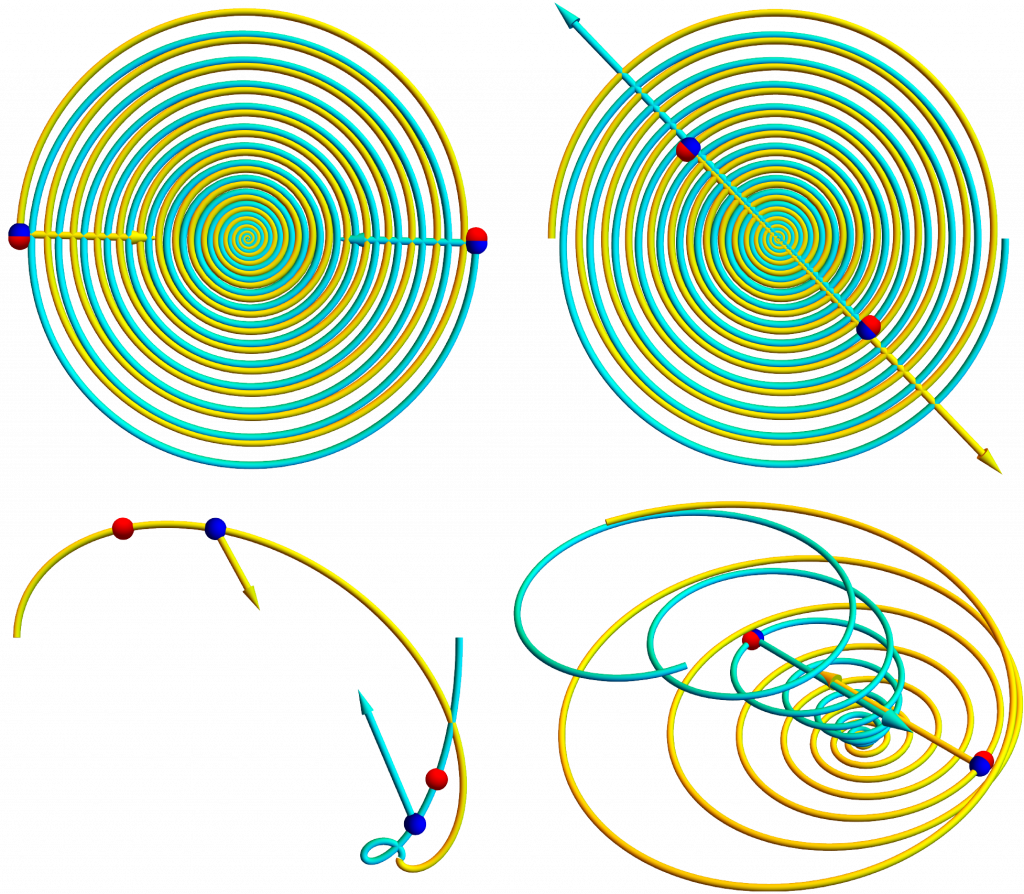Mathematica simulations of interacting charged particle including magnetic, radiation, and time-delay effects. Blue dots mark present positions, and red dots mark retarded positions. Arrows represent force pairs, which are typically not “equal but opposite”.

## Wooster Physics Alumni at Kent Displays

Three Wooster physics alumni who all work at Kent Displays, Inc. returned to campus last Thursday to share some info about the physics of liquid crystals as well as some of their personal journeys to Wooster and beyond.

See more information over at the NEWS page on the Wooster Physics website!

## Triple EVA

Since the mid 1960s, all space walks or extra-vehicular activities (EVAs) have involved just one or two astronauts — except once. In May 1992, on the STS-49 mission, the crew of the space shuttle Endeavour was attempting to rescue a stranded communications satellite, Intelsat 603. After repeated two-person EVAs failed to capture the satellite, NASA and the crew improvised the world’s first (and so far only) 3-person EVA. Anchored in the shuttle’s cargo bay, astronauts Richard Hieb, Thomas Akers, and Pierre Thuot simultaneously grabbed the large spinning Intelsat by hand. The shuttle crew later attached Intelsat 603 to a live rocket motor and released it, enabling the satellite to reach its desired geostationary orbit, where it successfully operated for 23 years.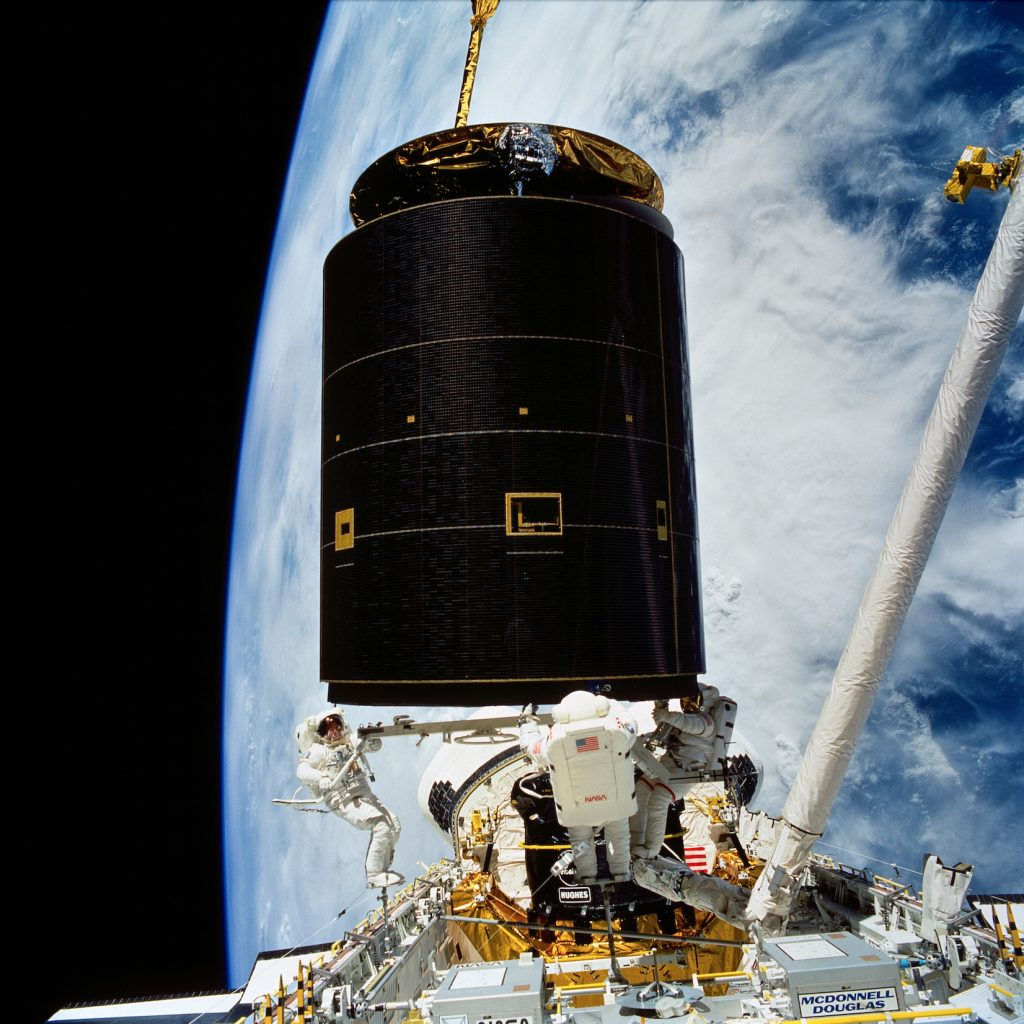During the world’s first (and so far only) 3-person EVA, space shuttle astronauts salvage a stranded communications satellite. (1992 May 13, NASA.)

## 5-Color Theorem

On 1852 October 23, Francis Guthrie noticed that he needed only 4 colors to color the counties of England so no two bordering counties shared the same color. This works for any map, but only in 1976, and with the aid of a computer, did Kenneth Appel and Wolfgang Haken finally prove the 4-color theorem. Here I outline an accessible proof of the simpler 5-color theorem based on Percy Heawood‘s 1890 salvage of Alfred Kempe‘s 1879 flawed proof.

## Convert map to graph

Replace the map with a graph of $V$ vertices, $E$ edges, and $F$ faces. Represent the 5 colors by the numbers 1, 2, 3, 4, 5.## Euler characteristic

For connected planar graphs, the Euler characteristic $\chi = V-E+F=2$ is a topological invariant. The proof is by induction on the number of vertices. An isolated vertex $V = 1$ connects to no edges $E = 0$ but is surrounded by a single face $F = 1$ extending to infinity, so $\chi = 1-0+1=2$. Assume $\chi = 2$ for a graph of $V$ vertices, and extend it in one of two ways: add an edge and a vertex, so $\Delta \chi =1 - 1 + 0 = 0$, or add an edge connecting two existing vertices, so $\Delta \chi =0 - 1 + 1 = 0$. Either way, the Euler characteristic is invariably $\chi = 2.$## Twice edges is at least thrice faces

For simple planar graphs (with no loops or repeated edges), double the number of edges is at least triple the number of faces. To prove this, first consider a maximally triangulated graph where each face (including the exterior one) is bounded by 3 edges. Tripling the faces counts each edge twice, so $2E = 3F$. To reach a generic case, deleting any edge reduces this equality’s left side by 2 and reduces its right side by 3, so $2E \ge 3F$.## At least one vertex has no more than 5 edges

In simple planar graphs, there exists a vertex with 5 or fewer edges. The proof is by contradiction. If each vertex connects to 6 edges, then sextupling the vertices counts each edge twice, so $2E = 6V$. For the generic case, where each vertex connects to at least 6 edges, adding edges increase this equality’s left side without changing its right side, so $2E \ge 6V$ and $E \ge 3V.$Use this inequality to eliminate vertices $V$ from the tripled Euler characteristic $3\chi = 3V-3E+3F = 6$ and get $E-3E+3F\ge 6$, so $3F - 6\ge 2E \ge 3F$ by the above twice-thrice inequality. But $3F - 6\ge 3F$ implies $- 6\ge 0$, which is a contradiction.

## 5-color theorem

Every map is 5-colorable. The proof is by induction on the number of vertices of the corresponding graphs. A single $V = 1$ vertex is trivially colored by a single color. Assume all $V > 1$ graphs are 5-colorable and consider a $V+1$ graph. Delete the vertex with the fewest edges, which must be 5 or less, and the resulting graph is 5-colorable. Restore the vertex. If it is connected to 4 or less edges, color it differently than its neighbors.If the restored vertex is connected by 5 edges, consider nonadjacent neighbor vertices $V_1$ and $V_4$ colored by colors 1 and 4. If the subgraph of vertices colored 1 and 4 is disconnected and $V_1$ and $V_4$ are in different components, interchange the 1 and 4 colors in the component including $V_1$ and color the restored vertex 1.Else if $V_1$ and $V_4$ are in the same component of the subgraph, a Kempe chain barrier separates the subgraph connecting $V_3$ and $V_5$, so  interchange the 3 and 5 colors in one component and color the restored vertex 5.## Compton Generator

Long before he won the Nobel Prize in Physics, and while still a Wooster undergraduate, Arthur Compton realized a third way to demonstrate Earth’s spin (after pendulums and gyroscopes).

Compton reported his results in a manuscript submitted to the journal Science on 1913 January 13 and published as “A Laboratory Method of Demonstrating the Earth’s Rotation”, Arthur Holly Compton, Physical Laboratory, University of Wooster, Science, 1913 May 23, volume 37, issue 960, pages 803-806.

Compton’s generator is nowadays often used as a text book example of the $\vec F_C = 2 m\, \vec v \times \vec \omega$ Coriolis pseudo-force deflecting the fluid in a circular tube as it is flipped in Earth’s rotating reference frame, which is analogous to the $\vec F_B = q\, \vec v \times \vec B$ magnetic force deflecting a moving charge. However, an inertial perspective is simpler. After viscosity has damped the fluid motion relative to Earth, the fluid farther from the rotation axis is moving faster relative to distant stars, so quickly flipping the ring reverses the speed gradient and induces transient circulation.

Flipping a wire loop in a magnetic field generates transient electric current like flipping Compton’s ring in a spinning reference frame generates transient fluid flow, where the magnetic field plays the role of the angular velocity; indeed, the former is an electric generator and the latter is a motion generator!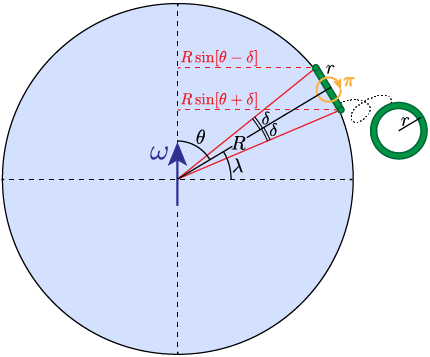Flipping Compton’s ring π = 180° causes the stationary fluid inside to circulate, generating motion and revealing Earth’s spin.

As in the above figure, assume Earth has radius $R$ and angular speed $\omega$. Assume the ring is at co-latitude $\theta$ and subtends an angle $2\delta$ from Earth’s center. Then the spread in the fluid’s inertial speeds is

$$\delta v = \omega R \sin[ \theta + \delta] - \omega R \sin[\theta - \delta] = 2\omega R \cos \theta\sin \delta.$$

If the ring’s radius $r \ll R$, then

$$1 \gg r / R \approx \delta \approx \sin\delta,$$

and so

$$\delta v = 2 \omega r \cos \theta = 2 \omega r \sin \lambda,$$

where $\lambda$ is the latitude. As checks, $\delta v = 0$ when $\lambda = 0$ (straddling the equator), and $\delta v = 2\omega r$ when $\lambda = \pi/2$ (straddling the north pole).

For Earth’s $\omega = 2\pi/\text{day}$ angular speed, flipping a quiescent $r = 1~\text{m}$ ring at $\lambda = \pi/4$ mid latitude generates an initial fluid speed $\delta v = 0.1~\text{mm/s}$. Compton amplified this motion by using a microscope to view the fluid through a window in a constriction of the tube.

## Analemma

Photograph the sky at the same time each day for a year and Sun will appear to execute a figure-8 path called an analemma, which is often inscribed on Earth globes and can be used as an almanac, as by Tom Hanks‘ character Chuck Nolan in the movie Cast Away.

The 2D animation below illustrates the formation of a 1D back-and-forth analemma for Planet whose spin axis is perpendicular to its orbital plane. Planet’s year divides into 7 equal sidereal days (when red arrow observer points left), 6 equal mean solar days  (when white ray from Sun crosses yellow lines), and 6 unequal apparent solar days (when red arrow points along white ray toward Sun).

Planet’s rotation (spin), which is constant, and eccentric revolution (orbit), which is faster nearer Sun where gravity is stronger, cause mean solar days to be nonuniformly distributed about the orbit, dense near aphelion (far point at left) and sparse at perihelion (near point at right). For an equatorial observer who experiences noon at perihelion, mean noon (when yellow dots record Sun’s direction) precedes apparent noon during the orbital top half and succeeds apparent noon during the orbital bottom half, with the constant rotation “falling behind” during the fast orbital right half and “getting ahead” during the slow orbital left half.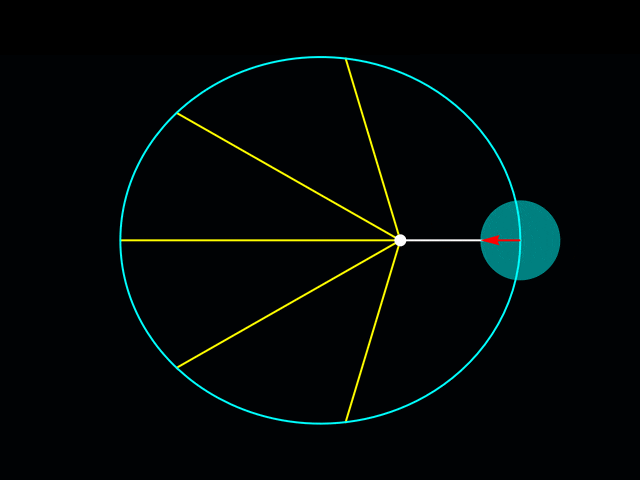Formation of a back-and-forth analemma (yellow dots) for an un-tilted planet in an eccentric orbit.
(You may need to click to see the animation.)

The 3D animation below (with a wide-angle perspective) illustrates the formation of a 2D figure-8 analemma for an oblique Planet whose spin axis is tilted 45° downward. Planet’s year divides into 40 equal sidereal days, 39 equal mean solar days, and 39 unequal apparent solar days. The constant tilt and elliptical orbit cause an equatorial observer to oscillate above and below Sun. For an equatorial observer who experiences noon at perihelion, mean noon (when yellow dots record Sun’s direction) precedes apparent noon, with Sun above the equator, during the orbital top half and succeeds apparent noon, with Sun below the equator, during the orbital bottom half, forming a figure 8. (In practice, the precise shape and orientation of analemmas vary with time of day and latitude.)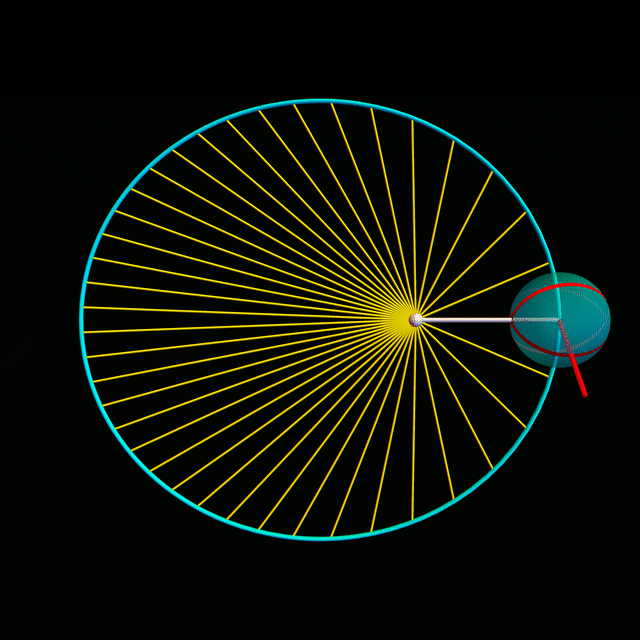Formation of a figure-8 analemma (yellow dots) for a tilted planet in an eccentric orbit. Showing the planet at mean solar day increments reduces bandwidth but hides most of its spin.
(You may need to click to see the animation.)